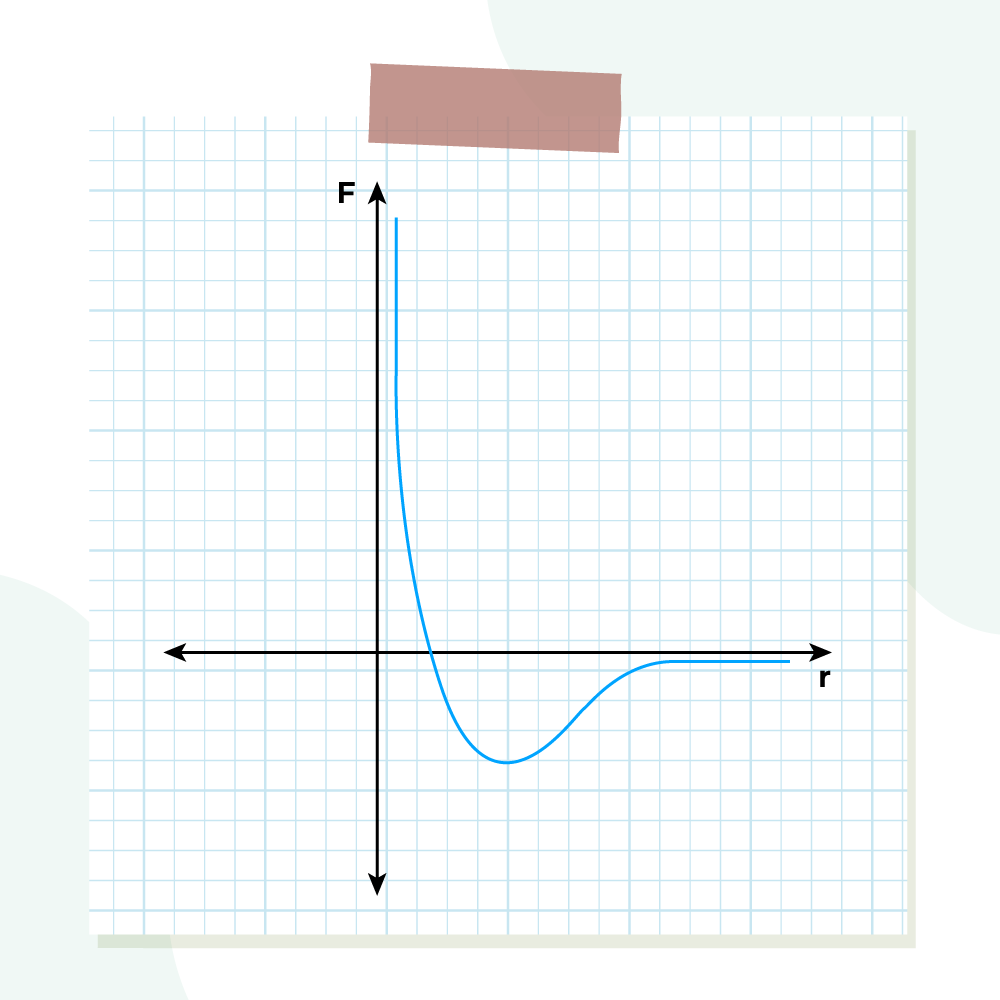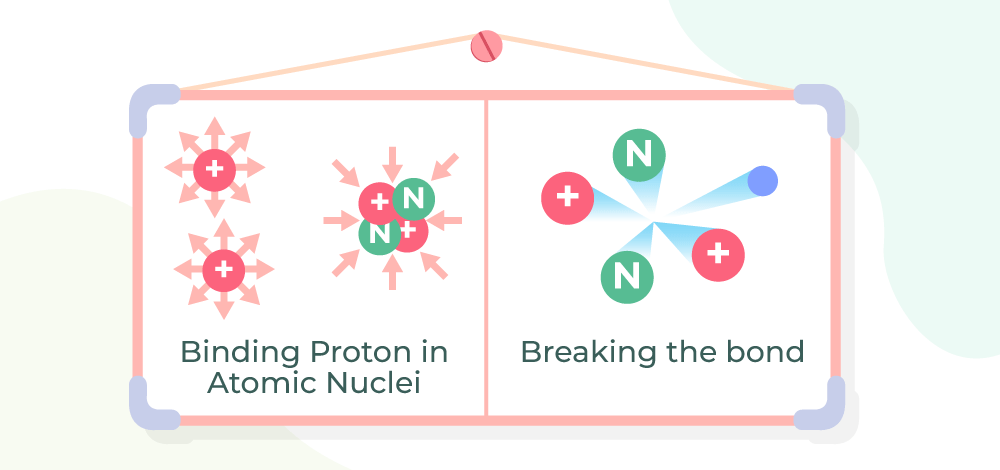GFG App
Open AppBrowser
Continue

Nuclear forces, strong nuclear force, and weak nuclear force are two of the four fundamental forces of nature other than electromagnetic and gravitational forces. Unlike Coulomb’s Law or Newton’s Law of Gravitation, there is no simple mathematical way to describe nuclear forces. Nuclear forces are one of the strongest forces of nature.

According to the standard model nuclear forces arise due to the exchange of gluons. Since nucleons are made up of quarks, i.e., neutron (udd) and proton (uud), where u and d denote up and down quarks, respectively. The exchange of quarks between the neutron and proton keeps them intact inside the nucleus.

## Fundamental Forces in Nature

There are four fundamental forces in nature which are,

• Gravitational Force
• Electromagnetic Force
• Strong Nuclear Force
• Weak Nuclear Force

Strong Nuclear Forces keep the nucleons together despite having a similar charge. Now, let’s learn about the Nuclear Force in detail in this article.

## What is Nuclear Force?

The nuclear forces exist inside the nucleus, i.e., the nuclear force exists between protons and neutrons and holds them together inside the nucleus.

Nuclear force exists between neutrons and neutrons, neutrons and protons, and protons and protons. As we know according to Coulomb’s Law same charges repel each other and protons are made of similar charges but still inside a nucleus protons are held together even though they repel each other, this is because of Nuclear force. The nuclear force is one of the strongest forces in nature hence it overcomes, the electrostatic force inside the nucleus and keeps it intact.

The nuclear forces are further classified into two categories which are discussed below,

## Types of Nuclear Force

On the basis of intensity nuclear force is further classified as,

• Strong Nuclear Force
• Weak Nuclear Force

### Strong Nuclear Force

Strong nuclear force is the force that acts between particles such as quarks, and other. It is the strongest force out of the four fundamental forces of interaction.  Two similar charges repel each other because of the Coulomb’s force, but in a nucleus, strong nuclear force overcomes the Colombian forces and keeps protons and protons together inside the nucleus. If the strong nuclear forces that bind protons and neutrons in an atom break, high-energy photons are released. Some of the properties of this force are,

• Strong nuclear forces are responsible for holding the nuclei of atoms stable.
• They are about 106 times greater than the weak nuclear force.
• They work at a distance of less than 10-15 m.

The magnitude of Strong Nuclear Force is far greater than the electromagnetic and gravitational force.

### Weak Nuclear Force

Weak nuclear force is involved in the particle where radioactive decay occurs. They change neutrons into protons in the process of nuclear decay. Some of the properties of this force are

• They exist between subatomic particles involving radioactive decay.
• They are neither attractive nor repulsive.

## Properties of Nuclear Forces

Various properties of Nuclear forces are discussed below,

### Nuclear Force is the strongest Fundamental Force

The magnitude of the nuclear force between two protons is 100 times Coulomb’s electrostatic repulsive force and 1036 times the gravitational attractive force. The nuclear force is stronger than Coulomb’s repulsive force and is able to keep the protons bound in a very small nucleus.

### Nuclear force is Attractive Force

Nuclear force is attractive in nature and the variation of the potential energy of the nucleus with the distance (r) between the nucleons is shown in the figure below,The graph in the figure reveals Potential energy is released at a distance of ro (≈ 0.8 fm). When the distance between two nucleons is greater than ro, the nuclear forces are attractive. As the distance between them decreases and is smaller than ro this force becomes repulsive and becomes minimum (≈ 0.8 fm) and increases rapidly which avoids the collapsing of the nucleus.

### Nuclear Force is Charge Independent

The interaction between two nucleons is independent of whether one or both nucleons have a charge on them. In other words, the nuclear force between Proton-Proton (p-p)proton-neutron (p-n), and neutron-neutron (n-n)is the same, so these forces are charge independent.

### Nuclear Force is Short-Range Force

The nuclear forces between two nucleons exist only when the distance between nucleons is comparable to the size of the nucleus i.e. of the order of 10-15. These forces cease to act as the distance between two nucleons exceeds 10-15. Moreover, a nucleon can interact with only its neighboring nucleons just as an atom in solid form bonds only with the surrounding atoms. Thus, these forces are short-range forces.

### Nuclear Force is Exchange Force

Nuclear forces are due to the exchange of π mesons between the nucleons, so they are called exchange forces. The force between two nucleons does not act along the line joining their centers and is therefore called non-Central force.

### Nuclear force is Spin-Dependent

It has been observed that the nuclear force between nucleons having parallel spins is greater than the force between nucleons having anti-parallel spins. Thus they are spin-dependent.

## Examples of Nuclear Forces

As previously mentioned, the most obvious example of Nuclear Force is the binding of protons, which are naturally repulsive due to their positive charge.

• Nuclear Force is responsible for the working of the nuclear reactor and nuclear weapons.
• Strong nuclear force is responsible for the stability of the nucleus of an atom.
• Weak nuclear force is responsible for radioactive decay.

## Nuclear Stability

Nuclear Stability can be explained, by three forces interacting with each other inside a nucleus.

• The main force responsible for the stability of the nucleus is the Strong Nuclear Force, which is responsible for nucleus cohesiveness by pushing the different nucleons together and is also responsible for the generation of alpha radiation.
• Electromagnetic Repulsion force acts between protons, but its magnitude is far less than the strong nuclear force.
• The third of these is the ‘weak‘ force, which works inside individual nucleons and can occasionally result in the change of a neutron into a proton (or vice versa), accompanied by the production of beta radiation.

The image shown below shows the nuclear stability and its bond breakage.## FAQs on Nuclear Force

### Question 1: What is the Nuclear Force?

The nuclear force is the force which is responsible for the stability of the nucleus. They are the strongest fundamental forces.

### Question 2: What is a Weak Nuclear Force?

Weak nuclear forces are the forces that are responsible for the radioactive decay of the nucleus. They result in nuclear decay, such as beta decay.

### Question 3: What is a strong nuclear force?

Strong nuclear forces are the forces that are responsible for the stability of the nucleus, they are the force that acts between nucleons. They are attractive forces.

### Question 4: Which of the following Particle are responsible for the Nuclear Force?

According to the standard model gluons (exchange particles between the quarks) are the fundamental particles which are responsible for the nuclear force.